omG8HI9LSKFW6lhCj8prTs0Z6lUhdOT9Jhi1Sf4m

# Difference Impedance and Admittance AC Electric Circuits

Impedance sounds strange for people who do not study electric circuits in advanced level. Impedance is often used in ac electric circuit analysis as well as resistance in dc electric circuit. The difference between these two is, impedance has magnitude and phase, while resistance only has magnitude.
Make sure to read what is ac circuit first.

From the previous post about sinusoidal and phasor, we knew the voltage-current relations for three passive elements of R, L, and C as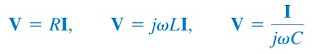(1)
These equations may be written in terms of the ratio of the phasor voltage to the phasor current as(2)
From these three expressions, we obtain Ohm's law in phasor form for any type of element as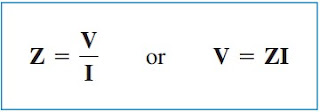(3)
where Z is a frequency-dependent quantity known as impedance, measured in Ω.
The impedance Z of a circuit is the ratio of the phasor voltage V to the phasor current I, measured in ohms (Ω)
The impedance shows the opposition that the circuit exhibits to the flow of sinusoidal current. Although the impedance is the ratio of two phasors, it is not a phasor, because it does not correspond to a sinusoidally varying quantity.

The impedance of resistors, inductors, and capacitors can be readily obtained from Equation.(2). Table.1 gives a summary of their impedances.Table.1
Looking from the table, we notice that ZL = jωL and ZC = -j/ωC. Consider two extreme cases of angular frequency. When ω = 0 (i.e., for dc sources), ZL = 0 and ZC → ∞, confirming what we already know - that the inductor acts like a short circuit, while the capacitor acts like an open circuit.

When ω→ ∞ (i.e., for high frequencies), ZL → ∞ and ZC = 0, showing that the inductor is an open circuit, while the capacitor is a short circuit as can be seen in Figure.(1).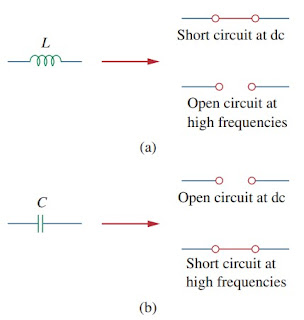Figure 1. Equivalent circuits at dc and high frequencies: (a) inductor, (b) capacitor
As a complex quantity, the impedance may be written in rectangular form as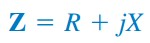(4)
where R = Re Z is the resistance and X = Im Z is the reactance. The reactance X may be negative or positive. We may say that the impedance is inductive when X is positive or capacitive when X is negative.

Hence, impedance Z = R + jX is said to be inductive or lagging since current lags voltage, while impedance Z = R - jX is capacitive or leading since current leads voltage. All three impedance, resistance, and reactance are measured in ohms. The impedance may also be expressed in polar form as(5)
Comparing Equations.(4) and (5), we conclude that(8)
It is sometimes convenient to work with the reciprocal of impedance, known as admittance.
The admittance Y is the reciprocal of impedance, measured in siemens (S).
The admittance Y of an element (or a circuit) is the ratio of the phasor current through it to the phasor voltage across it, or(9)
The admittance of resistors, inductors, and capacitors can be obtained from Equation.(2). They also summarized in Table.(1).

As a complex quantity, we may write Y as(10)
where G = Re Y is called the conductance and B = Im Y is called the susceptance. Admittance, conductance, and susceptance are all expressed in the unit of siemens (or mhos). From Equations.(4) and (10),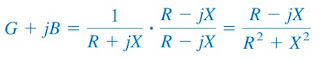(12)
Equating the real and imaginary parts give(13)
showing that G ≠ 1/R as it is in resistive circuits. Of course, if X = 0, then G = 1/R.

For better understanding let us review the example below :
1. Find v(t) and i(t) in the circuit in Figure.(2)
From the voltage source 10 cos 4t, ω = 4,(1.2)
Converting I and V in Equations.(1.1) and (1.2) to the time domain results
Notice that i(t) leads v(t) by 90o as expected.

Have you understood what is impedance? Don't forget to share and subscribe! Happy learning!
Reference:  Fundamentals of electric circuits by Charles K. Alexander and Matthew N. O. Sadiku

Untuk Bahasa Indonesia baca Rumus Impedansi dan Admitansi Rangkaian AC
Related Posts
SHARE